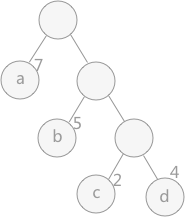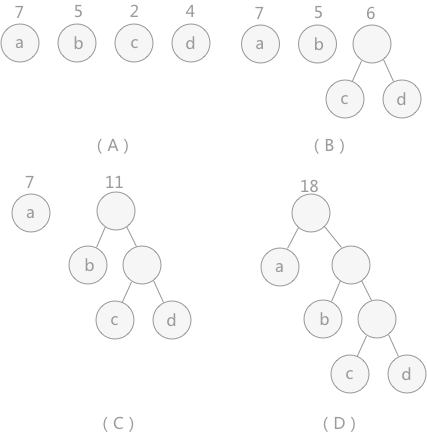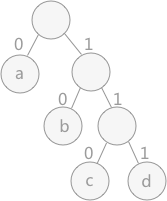## 与哈夫曼树相关名词WPL = 7 * 1 + 5 * 2 + 2 * 3 + 4 * 3

## 构建哈夫曼树

1. 在 n 个权值中选出两个最小的权值，对应的两个结点组成一个新的二叉树，且新二叉树的根结点的权值为左右孩子权值的和；
2. 在原有的 n 个权值中删除那两个最小的权值，同时将新的权值加入到 n–2 个权值的行列中，以此类推；
3. 重复 1 和 2 ，直到所以的结点构建成了一棵二叉树为止，这棵树就是哈夫曼树。• （A）给定了四个结点a，b，c，d，权值分别为7，5，2，4；
• 第一步如（B）所示，找出现有权值中最小的两个，2 和 4 ，相应的结点 c 和 d 构建一个新的二叉树，树根的权值为 2 + 4 = 6，同时将原有权值中的 2 和 4 删掉，将新的权值 6 加入；
• 进入（C），重复之前的步骤。直到（D）中，所有的结点构建成了一个全新的二叉树，这就是哈夫曼树。

## 哈夫曼树的节点结构

 ``````1 2 3 4 5 `````` ``````//哈夫曼树结点结构 typedef struct { int weight;//结点权重 int parent, left, right;//父结点、左孩子、右孩子在数组中的位置下标 }HTNode, *HuffmanTree; ``````

### 哈夫曼树中的查找算法

• 如果比两个结点中较小的那个还小，就保留这个结点，删除原来较大的结点；
• 如果介于两个结点权重值之间，替换原来较大的结点；

 `````` 1 2 3 4 5 6 7 8 9 10 11 12 13 14 15 16 17 18 19 20 21 22 23 24 25 26 27 28 29 30 31 32 33 34 35 36 37 38 39 40 41 42 43 44 45 46 47 48 `````` ``````//HT数组中存放的哈夫曼树，end表示HT数组中存放结点的最终位置，s1和s2传递的是HT数组中权重值最小的两个结点在数组中的位置 void Select(HuffmanTree HT, int end, int *s1, int *s2) { int min1, min2; //遍历数组初始下标为 1 int i = 1; //找到还没构建树的结点 while(HT[i].parent != 0 && i <= end){ i++; } min1 = HT[i].weight; *s1 = i; i++; while(HT[i].parent != 0 && i <= end){ i++; } //对找到的两个结点比较大小，min2为大的，min1为小的 if(HT[i].weight < min1){ min2 = min1; *s2 = *s1; min1 = HT[i].weight; *s1 = i; }else{ min2 = HT[i].weight; *s2 = i; } //两个结点和后续的所有未构建成树的结点做比较 for(int j=i+1; j <= end; j++) { //如果有父结点，直接跳过，进行下一个 if(HT[j].parent != 0){ continue; } //如果比最小的还小，将min2=min1，min1赋值新的结点的下标 if(HT[j].weight < min1){ min2 = min1; min1 = HT[j].weight; *s2 = *s1; *s1 = j; } //如果介于两者之间，min2赋值为新的结点的位置下标 else if(HT[j].weight >= min1 && HT[j].weight < min2){ min2 = HT[j].weight; *s2 = j; } } } ``````

## 哈夫曼编码1. 从叶子结点一直找到根结点，逆向记录途中经过的标记。例如，图中字符 c 的哈夫曼编码从结点 c 开始一直找到根结点，结果为：0 1 1 ，所以字符 c 的哈夫曼编码为：1 1 0（逆序输出）。

 `````` 1 2 3 4 5 6 7 8 9 10 11 12 13 14 15 16 17 18 19 20 21 22 23 24 25 26 27 28 29 30 31 `````` ``````//HT为哈夫曼树，HC为存储结点哈夫曼编码的二维动态数组，n为结点的个数 void HuffmanCoding(HuffmanTree HT, HuffmanCode *HC,int n){ *HC = (HuffmanCode) malloc((n+1) * sizeof(char *)); char *cd = (char *)malloc(n*sizeof(char)); //存放结点哈夫曼编码的字符串数组 cd[n-1] = '\0';//字符串结束符 for(int i=1; i<=n; i++){ //从叶子结点出发，得到的哈夫曼编码是逆序的，需要在字符串数组中逆序存放 int start = n-1; //当前结点在数组中的位置 int c = i; //当前结点的父结点在数组中的位置 int j = HT[i].parent; // 一直寻找到根结点 while(j != 0){ // 如果该结点是父结点的左孩子则对应路径编码为0，否则为右孩子编码为1 if(HT[j].left == c) cd[--start] = '0'; else cd[--start] = '1'; //以父结点为孩子结点，继续朝树根的方向遍历 c = j; j = HT[j].parent; } //跳出循环后，cd数组中从下标 start 开始，存放的就是该结点的哈夫曼编码 (*HC)[i] = (char *)malloc((n-start)*sizeof(char)); strcpy((*HC)[i], &cd[start]); } //使用malloc申请的cd动态数组需要手动释放 free(cd); } ``````
1. 从根结点出发，一直到叶子结点，记录途中经过的标记。例如，求图中字符 c 的哈夫曼编码，就从根结点开始，依次为：1 1 0。

 `````` 1 2 3 4 5 6 7 8 9 10 11 12 13 14 15 16 17 18 19 20 21 22 23 24 25 26 27 28 29 30 31 32 33 34 35 36 37 38 39 40 41 42 43 44 45 `````` ``````//HT为哈夫曼树，HC为存储结点哈夫曼编码的二维动态数组，n为结点的个数 void HuffmanCoding(HuffmanTree HT, HuffmanCode *HC,int n){ *HC = (HuffmanCode) malloc((n+1) * sizeof(char *)); int m=2*n-1; int p=m; int cdlen=0; char *cd = (char *)malloc(n*sizeof(char)); //将各个结点的权重用于记录访问结点的次数，首先初始化为0 for (int i=1; i<=m; i++) { HT[i].weight=0; } //一开始 p 初始化为 m，也就是从树根开始。一直到p为0 while (p) { //如果当前结点一次没有访问，进入这个if语句 if (HT[p].weight==0) { HT[p].weight=1;//重置访问次数为1 //如果有左孩子，则访问左孩子，并且存储走过的标记为0 if (HT[p].left!=0) { p=HT[p].left; cd[cdlen++]='0'; } //当前结点没有左孩子，也没有右孩子，说明为叶子结点，直接记录哈夫曼编码 else if(HT[p].right==0){ (*HC)[p]=(char*)malloc((cdlen+1)*sizeof(char)); cd[cdlen]='\0'; strcpy((*HC)[p], cd); } } //如果weight为1，说明访问过一次，即是从其左孩子返回的 else if(HT[p].weight==1){ HT[p].weight=2;//设置访问次数为2 //如果有右孩子，遍历右孩子，记录标记值 1 if (HT[p].right!=0) { p=HT[p].right; cd[cdlen++]='1'; } } //如果访问次数为 2，说明左右孩子都遍历完了，返回父结点 else{ HT[p].weight=0; p=HT[p].parent; --cdlen; } } } ``````

## 遍历哈夫曼树程序设计

 `````` 1 2 3 4 5 6 7 8 9 10 11 12 13 14 15 16 17 18 19 20 21 22 23 24 25 26 27 28 29 30 31 32 33 34 35 36 37 38 39 40 41 42 43 44 45 46 47 48 49 50 51 52 53 54 55 56 57 58 59 60 61 62 63 64 65 66 67 68 69 70 71 72 73 74 75 76 77 78 79 80 81 82 83 84 85 86 87 88 89 90 91 92 93 94 95 96 97 98 99 100 101 102 103 104 105 106 107 108 109 110 111 112 113 114 115 116 117 118 119 120 121 122 123 124 125 126 127 128 129 130 131 132 133 134 135 136 137 138 139 140 141 142 143 `````` ``````#include #include #include //哈夫曼树结点结构 typedef struct { int weight;//结点权重 int parent, left, right;//父结点、左孩子、右孩子在数组中的位置下标 }HTNode, *HuffmanTree; //动态二维数组，存储哈夫曼编码 typedef char ** HuffmanCode; //HT数组中存放的哈夫曼树，end表示HT数组中存放结点的最终位置，s1和s2传递的是HT数组中权重值最小的两个结点在数组中的位置 void Select(HuffmanTree HT, int end, int *s1, int *s2) { int min1, min2; //遍历数组初始下标为 1 int i = 1; //找到还没构建树的结点 while(HT[i].parent != 0 && i <= end){ i++; } min1 = HT[i].weight; *s1 = i; i++; while(HT[i].parent != 0 && i <= end){ i++; } //对找到的两个结点比较大小，min2为大的，min1为小的 if(HT[i].weight < min1){ min2 = min1; *s2 = *s1; min1 = HT[i].weight; *s1 = i; }else{ min2 = HT[i].weight; *s2 = i; } //两个结点和后续的所有未构建成树的结点做比较 for(int j=i+1; j <= end; j++) { //如果有父结点，直接跳过，进行下一个 if(HT[j].parent != 0){ continue; } //如果比最小的还小，将min2=min1，min1赋值新的结点的下标 if(HT[j].weight < min1){ min2 = min1; min1 = HT[j].weight; *s2 = *s1; *s1 = j; } //如果介于两者之间，min2赋值为新的结点的位置下标 else if(HT[j].weight >= min1 && HT[j].weight < min2){ min2 = HT[j].weight; *s2 = j; } } } //HT为地址传递的存储哈夫曼树的数组，w为存储结点权重值的数组，n为结点个数 void CreateHuffmanTree(HuffmanTree *HT, int *w, int n) { if(n<=1) return; // 如果只有一个编码就相当于0 int m = 2*n-1; // 哈夫曼树总节点数，n就是叶子结点 *HT = (HuffmanTree) malloc((m+1) * sizeof(HTNode)); // 0号位置不用 HuffmanTree p = *HT; // 初始化哈夫曼树中的所有结点 for(int i = 1; i <= n; i++) { (p+i)->weight = *(w+i-1); (p+i)->parent = 0; (p+i)->left = 0; (p+i)->right = 0; } //从树组的下标 n+1 开始初始化哈夫曼树中除叶子结点外的结点 for(int i = n+1; i <= m; i++) { (p+i)->weight = 0; (p+i)->parent = 0; (p+i)->left = 0; (p+i)->right = 0; } //构建哈夫曼树 for(int i = n+1; i <= m; i++) { int s1, s2; Select(*HT, i-1, &s1, &s2); (*HT)[s1].parent = (*HT)[s2].parent = i; (*HT)[i].left = s1; (*HT)[i].right = s2; (*HT)[i].weight = (*HT)[s1].weight + (*HT)[s2].weight; } } //HT为哈夫曼树，HC为存储结点哈夫曼编码的二维动态数组，n为结点的个数 void HuffmanCoding(HuffmanTree HT, HuffmanCode *HC,int n){ *HC = (HuffmanCode) malloc((n+1) * sizeof(char *)); char *cd = (char *)malloc(n*sizeof(char)); //存放结点哈夫曼编码的字符串数组 cd[n-1] = '\0';//字符串结束符 for(int i=1; i<=n; i++){ //从叶子结点出发，得到的哈夫曼编码是逆序的，需要在字符串数组中逆序存放 int start = n-1; //当前结点在数组中的位置 int c = i; //当前结点的父结点在数组中的位置 int j = HT[i].parent; // 一直寻找到根结点 while(j != 0){ // 如果该结点是父结点的左孩子则对应路径编码为0，否则为右孩子编码为1 if(HT[j].left == c) cd[--start] = '0'; else cd[--start] = '1'; //以父结点为孩子结点，继续朝树根的方向遍历 c = j; j = HT[j].parent; } //跳出循环后，cd数组中从下标 start 开始，存放的就是该结点的哈夫曼编码 (*HC)[i] = (char *)malloc((n-start)*sizeof(char)); strcpy((*HC)[i], &cd[start]); } //使用malloc申请的cd动态数组需要手动释放 free(cd); } //打印哈夫曼编码的函数 void PrintHuffmanCode(HuffmanCode htable,int *w,int n) { printf("Huffman code : \n"); for(int i = 1; i <= n; i++) printf("%d code = %s\n",w[i-1], htable[i]); } int main(void) { int w = {2, 8, 7, 6, 5}; int n = 5; HuffmanTree htree; HuffmanCode htable; CreateHuffmanTree(&htree, w, n); HuffmanCoding(htree, &htable, n); PrintHuffmanCode(htable, n); return 0; } ``````